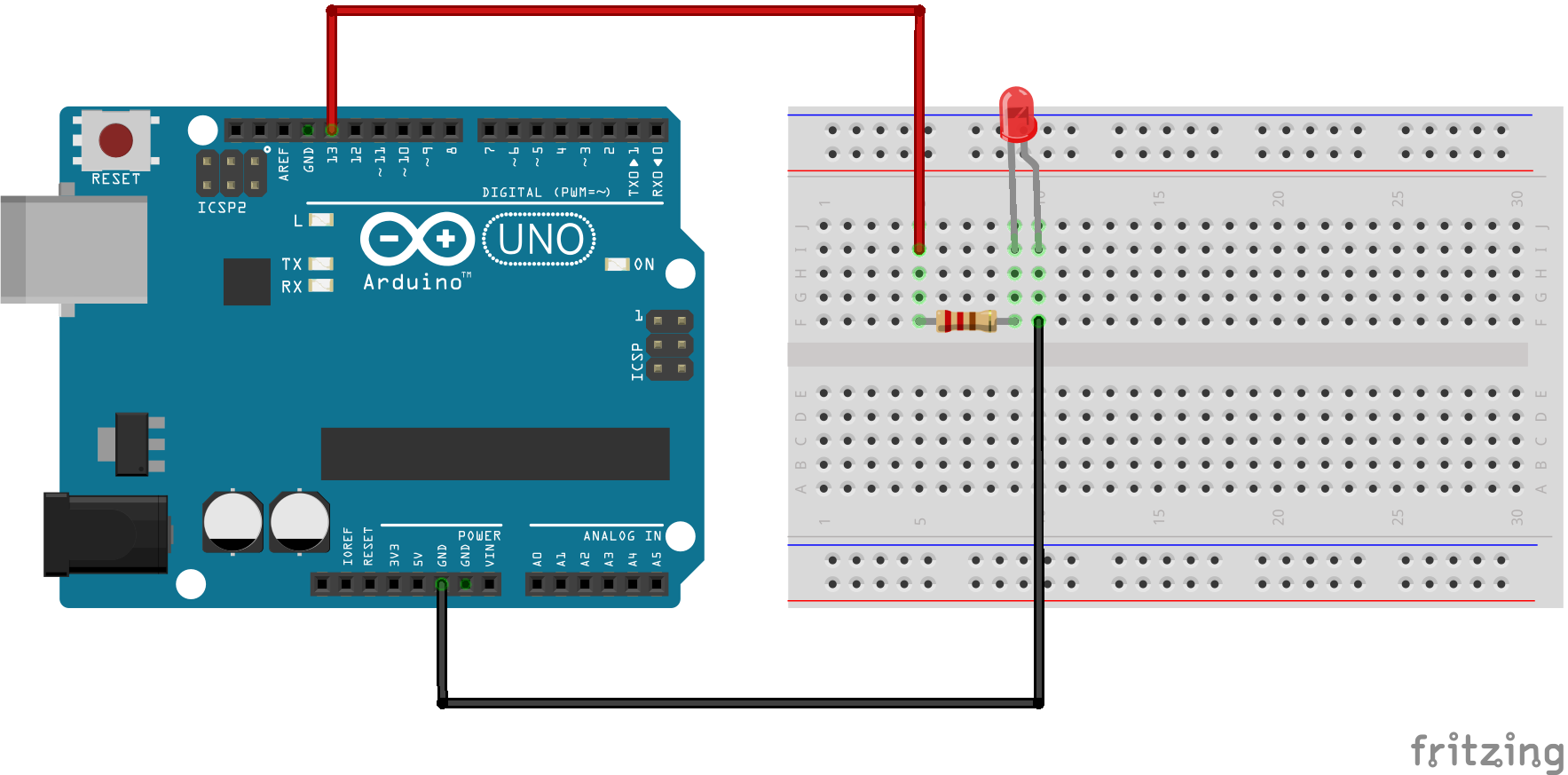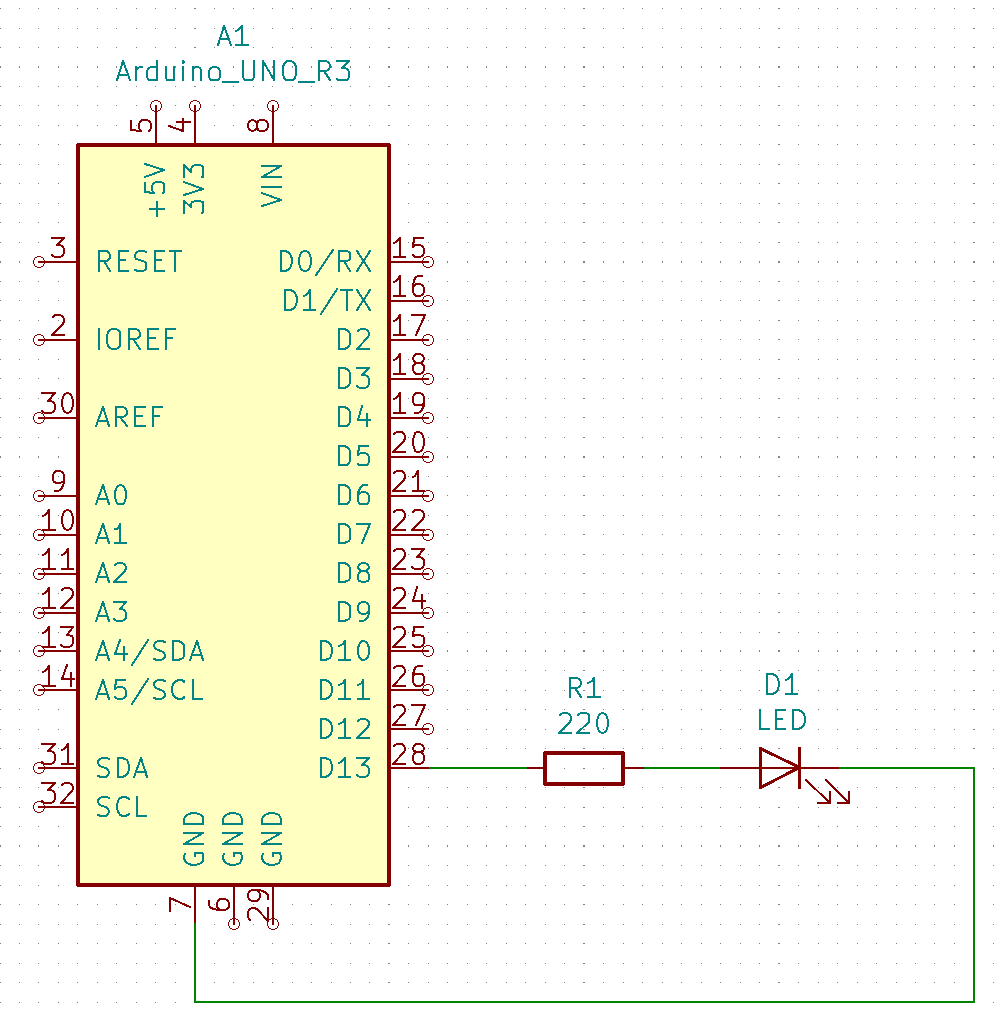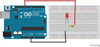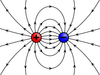## How to Blink an LED Using Arduino

This project is the "Hello World!" of Arduino. In this project, we are going to learn about physical outputs and two electronic components, while performing the most straightforward project in the electronics sea of projects.

## 1.0 Hardware Required

• Arduino Board
• LED
• 220Ω Resistor

## 2.0 Background Knowledge

### 2.1 Series Circuits

In this project, components are connected in a series configuration. A series circuit provides only one path for current between two points so that the current is the same through each series component. 

### 2.2 Light Emitting diode - LED

A light-emitting diode (LED) is a semiconductor light source that emits light when current flows through it. 

### 2.3 Resistor

A resistor is a passive two-terminal electrical component that implements electrical resistance as a circuit element. In electronic circuits, resistors are used to reduce current flow, adjust signal levels, to divide voltages, bias active elements, and terminate transmission lines, among other uses. 

## 3.0 Circuit

To build the physical circuit for this project follow figure 1. Note that an Arduino Uno has been used in this circuit. However, any Arduino can be used.Figure 1 - Visual Circuit

1. Place resistor and LED on the breadboard.
2. Connect one leg of the resistor to digital pin 13 of the Arduino
3. Connect another leg of the resistor to the log leg of the LED (the positive leg, called anode)
4. Connect the short leg of the LED (the negative leg, called cathode) to the ground (called GND) 

## 4.0 Schematic## 5.0 Code

After you build the circuit plug your Arduino board into your computer, start the Arduino Software (IDE) and enter the code below. 

## 7.0 References

 T. L. Floyd, Principles of Electric Circuits, New Jersey: Pearson Education, Inc, 2010.

 "Light-emitting diode," wikipedia, [Online]. Available: https://en.wikipedia.org/wiki/Light-emitting_diode. [Accessed 20 05 2019].

 "Resistor," wikipedia, [Online]. Available: https://en.wikipedia.org/wiki/Resistor. [Accessed 20 05 2019].

 SM, "Blink," Arduino CC, 28 07 2015. [Online]. Available: https://www.arduino.cc/en/Tutorial/Blink. [Accessed 20 05 2019].

## Related Posts##### How to Fade an LED Using Arduino
In this example, we use the analogWrite() function to fade an LED on and off. analogWrite() uses pulse with modulation (##### What Is An Electrical Charge?
The charge of an electron and that of a proton are equal in magnitude. Electrical charge is an electrical property of ma